# SUMIF function in Excel sheets explained

Originally published at: SUMIF function in Excel sheets explained | ONLYOFFICE Blog

Among all the math and trigonometry functions available in Excel, SUMIF is one of the most useful and frequently used. It sums up cells that correspond to the given criteria. Read this article to find out how this function works and how you can use it in your Excel spreadsheets.## What is the SUMIF function in Excel?

The SUMIF function belongs to a group of Excel functions that are used to summarize large data sets. When applied, SUMIF returns the sum of cells in a range that meet a certain condition, also referred to as criteria.

Criteria can include dates, numbers and text values. The SUMIF function is compatible with logical operators (>,<,<>,=) and supports wildcards. For example, the question mark (?) can replace any single character and the asterisk (*) can be used instead of any number of characters.

SUMIF has the following syntax:

=SUMIF(range, criteria, [sum_range])

As you can see, there are three arguments in the function. The first two are required, and the last one is optional:

• range: the range of cells that you want to apply the criteria to;
• criteria: the criteria which are used to determine the cells to be added;
• sum-range: the range to sum if the criteria are met. If omitted, the cells in the range argument are summed.

Please note that if your criteria contain text values or logical operators, they must be enclosed in double quotation marks. For example, “oranges” and “=20”. When it comes to cell references, they must be used without the quotation marks. If they are used with the quotation marks, they will be treated as text strings.

Now that you know the basics of the SUMIF function, it’s time to take a look at how it works in practice using ONLYOFFICE Spreadsheet Editor.

## How to insert the SUMIF function in Excel sheets

After opening your Excel spreadsheet in the ONLYOFFICE editor, you can insert SUMIF in two ways. First of all, you can access this function via the Home tab on the top toolbar. To do so, just click the Insert function button.After that, choose Additional from the drop-down list, and you will see the Insert function window that contains all the available functions classified by category. Enter SUMIF in the search box to find the required function and click OK.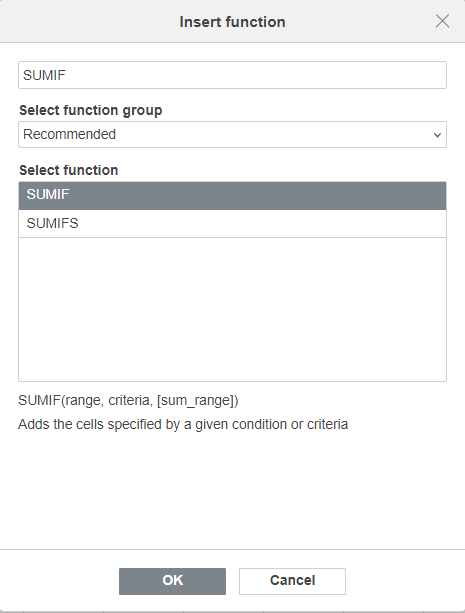Please note that you will also see the SUMIFS function. It’s similar to the SUMIF formula but implies multiple conditions. In other words, you can use it to find a sum of values based on multiple criteria.

Alternatively, you can add the SUMIF function via the Formula tab on the top toolbar. You just need to click Math and trigonometry and select SUMIF from the drop-down list.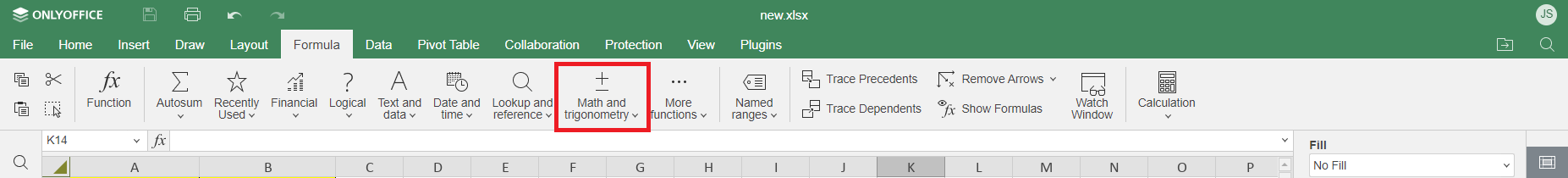No matter which method you prefer, you will see the Function arguments window. Here you need to specify the Range, Criteria and Sum_range (optional) arguments by entering the required values in the corresponding fields. When ready, click OK.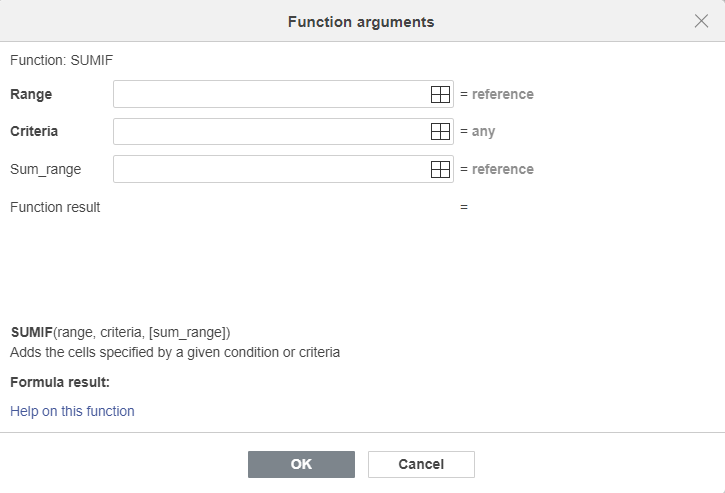To better understand the syntax of the SUMIF function and how it works, let’s refer to a real example.

## SUMIF formula example

Let’s consider the following example. In our Excel spreadsheet below, the table shows the total amount of revenue generated by our salespeople over a given period of time.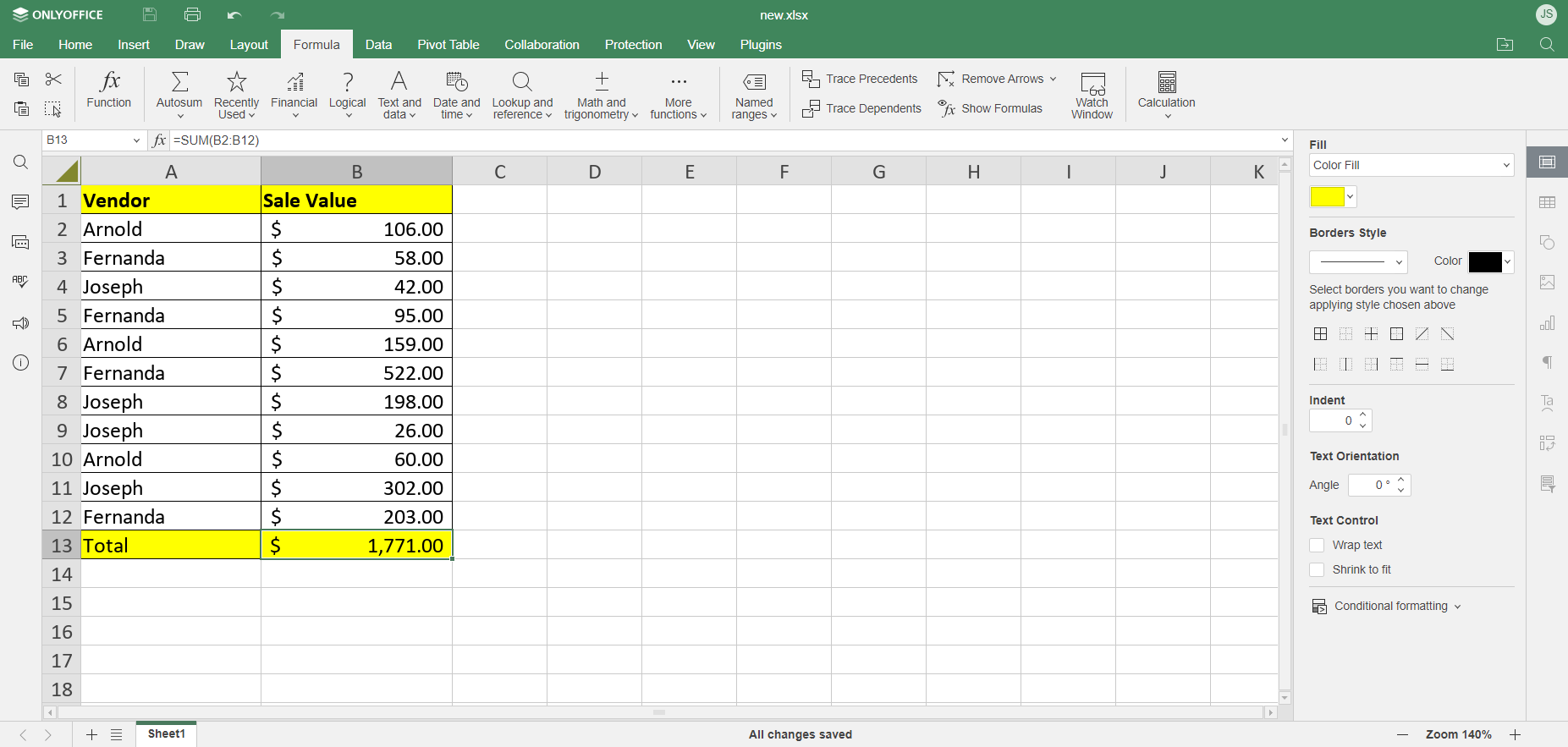As you can see, in our Sales team there are three employees: Joseph, Fernanda and Arnold. We know that the total sales of the three is \$1,771.00. But how much revenue did each of them generate? To find out, we resort to the SUMIF function.

To get started, we need to create another table with the name of each salesperson so that the SUMIF function can return the corresponding value for each of them.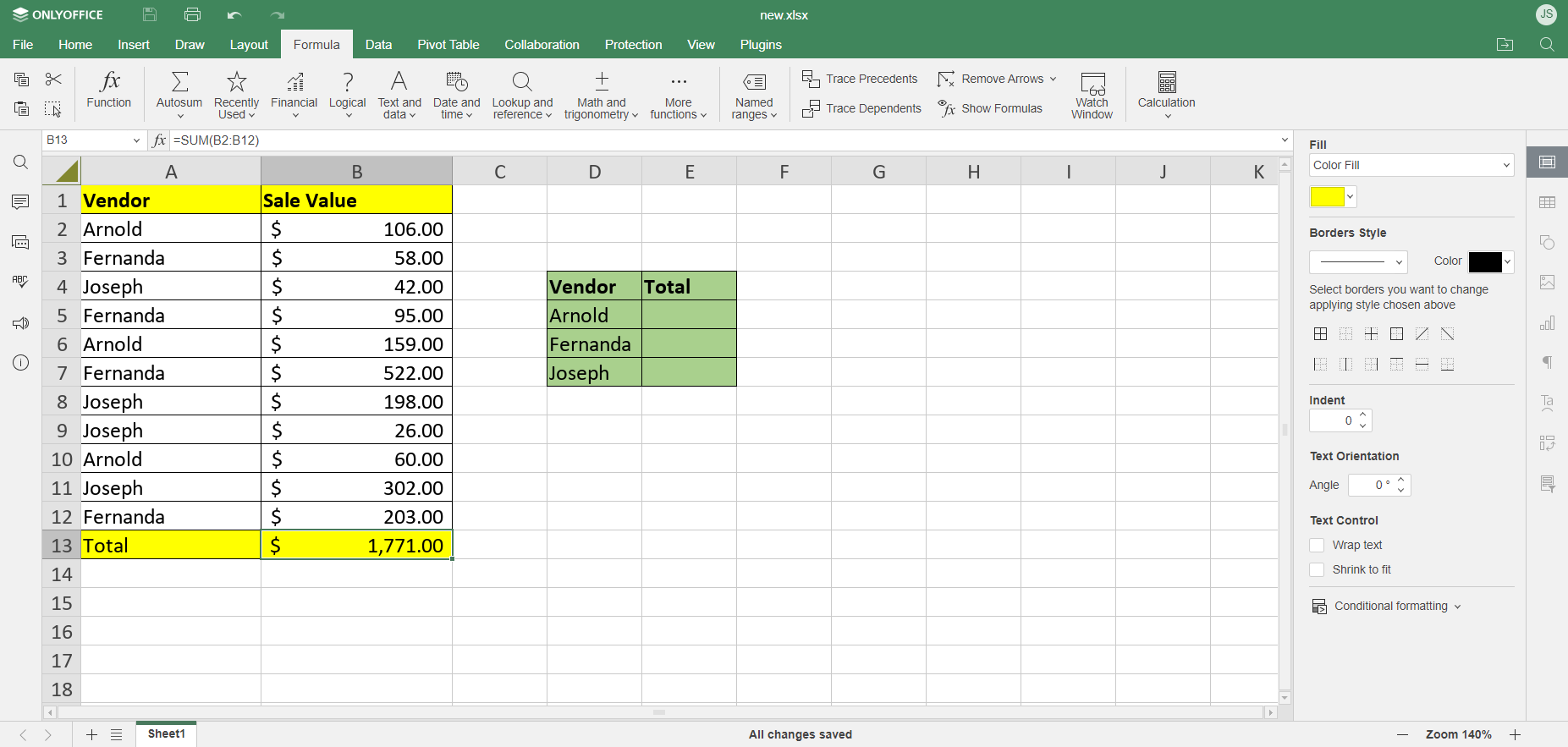The first salesperson on our list is Arnold. Let’s get a total of sales for him in Cell E5 by building a simple SUMIF formula. We need to access the required function using one of the methods above and specify the following arguments:

• Range — a list of vendors (A2:A12);
• Criteria — “Arnold” or a cell containing this name (D5);
• Sum_range — the sales amounts to be added up (B2:B12).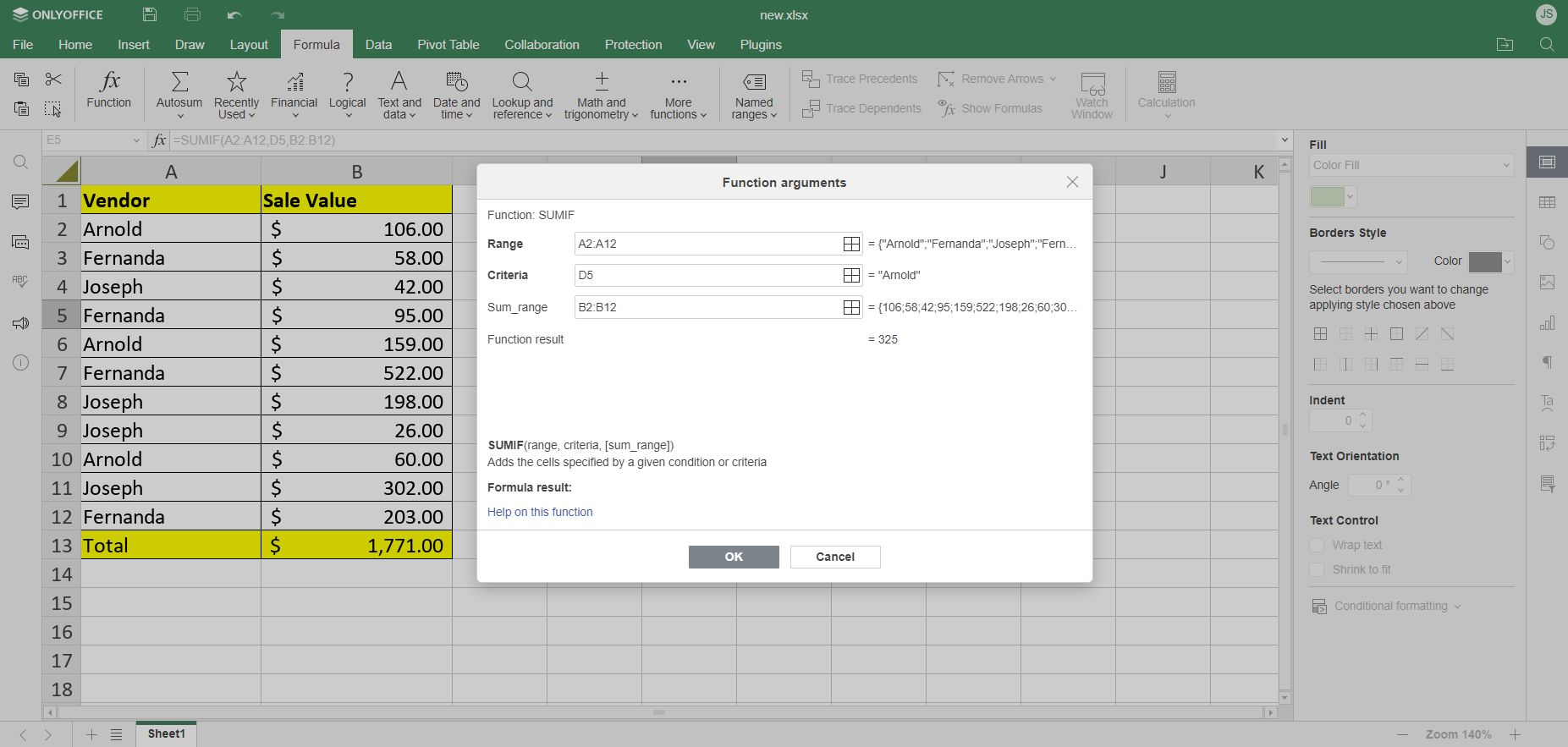Putting everything together, we get the following formula:

=SUMIF(A2:A12,D5,B2:B12)

Click OK to find out the total revenue generated by Arnold.Using the same algorithm, we calculate the performance of the other salespersons:

• Fernanda (E6): =SUMIF(A2:A12,D6,B2:B12)
• Joseph (E7): =SUMIF(A2:A12,D7,B2:B12)

If done correctly, we get the following table:Now we can see that Fernanda is the best salesperson over the given period of time with the highest revenue generated. We came to this conclusion with the help of the SUMIF function which made sense of a set of diverse data in our Excel spreadsheet.